# Phobrain

## Photo classification with TensorFlow For Poets: Part 2: Applying the models

Thanks to Google for making this easy-to-use package available.

Previously: Part 1: calculating the models.

Here I try the trained models on photos, to see if they can help me automate my manual keywording process (which is an aid in forming pairs of photos that are calculated to turn your mind inside-out, as an analytical replacement for materialism and to make me rich someday).

My keyword models answer questions like, what colors are prominent? Does a curve or angle seem like a major geometric component? Does art seem more like graffiti or a mural? Is one face prominent, or many? The holy grail is the as-many-keywords-as-possible model (27 keywords with at least 300 photos to train on per keyword), which one would like to describe any photo, given enough photos to train with. I try that with 3 successive orders of magnitude of optimization.

The main disappointment is false positives. E.g. it always finds a face (and never faces). Possibly that's due to lack of training data, and likely it would help a lot if the deeper net could be trained on my concepts, which might happen someday.

Next might be to train to distinguish day from night from indoors.

Refer to the previous page to see the model classes and how well each model fit. Classes scoring less than 0.1 per photo are often omitted below.

N: 15K photos, 400x400. Multiple keywords may apply to a given training photo. Tests were run on a 2012 laptop.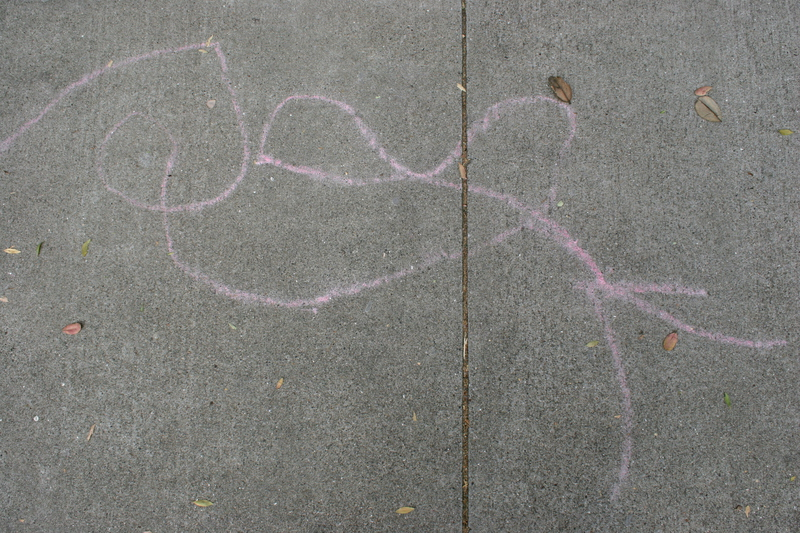ModelTop choices
Colorsgreen (score = 0.85230)
Countsarray (score = 0.91422)
Face/facesface (score = 0.91286)
Geom/no square, triangleradiate (score = 0.52076)
Geom: angular, curvecurve (score = 0.93541)
Geom: no angular, curveradiate (score = 0.58888)
Graffiti/muralgraffiti (score = 0.97779)
Juxtaposeontop (score = 0.49517)
Cases gt300texture (score = 0.19945)
graffiti (score = 0.11281)
curve (score = 0.09692)
Cases gt300 long optgraffiti (score = 0.39141)
array (score = 0.09557)
texture (score = 0.07082)
Cases gt300 longest optarray (score = 0.35357)
graffiti (score = 0.30821)
red (score = 0.02419)
pink (score = 0.01227)
texture (score = 0.01067)ModelTop choices
Colorsyellow (score = 0.92534)
Countsarray (score = 0.73452)
Face/facesface (score = 0.96524)
triangle (score = 0.21664)
curve (score = 0.15887)
circle (score = 0.10530)
angular (score = 0.09116)
square (score = 0.03195)
Geom/no square, triangleradiate (score = 0.63885)
Geom: angular, curveangular (score = 0.59718)
curve (score = 0.40282)
Geom: no angular, curveradiate (score = 0.55076)
triangle (score = 0.33511)
circle (score = 0.09196)
square (score = 0.02218)
Graffiti/muralmural (score = 0.66006)
graffiti (score = 0.33994)
Juxtaposejuxtapose (score = 0.45181)
opposite (score = 0.26858)
Cases gt300yellow (score = 0.31545)
Cases gt300 long optyellow (score = 0.72372)
Cases gt300 longest optyellow (score = 0.40654)
reflection (score = 0.34279)
orange (score = 0.05169)
face (score = 0.04552)
curve (score = 0.03867)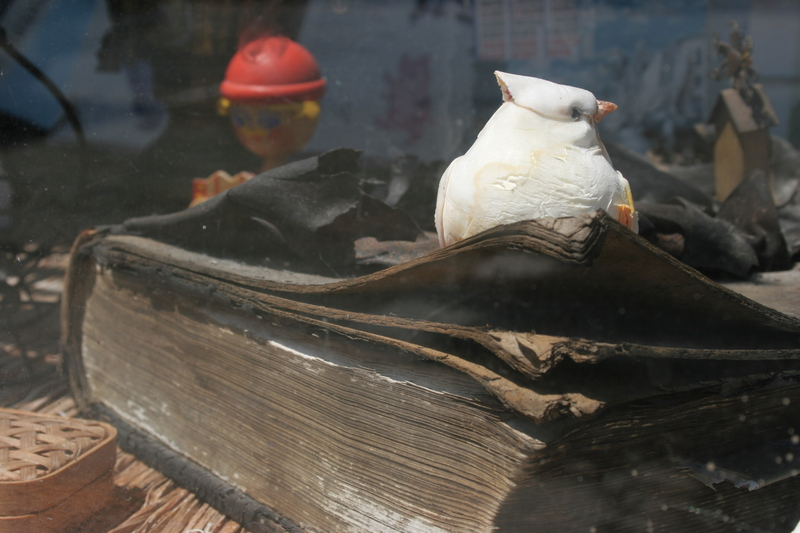ModelTop choices
Colorsyellow (score = 0.57743)
red (score = 0.36051)
Countstrio (score = 0.52385)
array (score = 0.26406)
pair (score = 0.21209)
Face/facesface (score = 0.74508)
faces (score = 0.25492)
angular (score = 0.23431)
triangle (score = 0.06652)
circle (score = 0.06065)
square (score = 0.01585)
Geom/no square curve (score = 0.32420)
angular (score = 0.25681)
Geom/no square, triangle angular (score = 0.36774)
curve (score = 0.29127)
Geom: angular, curveangular (score = 0.53799)
curve (score = 0.46201)
Geom: no angular, curveradiate (score = 0.73876)
triangle (score = 0.12957)
circle (score = 0.10584)
square (score = 0.02582)
Graffiti/muralmural (score = 0.50880)
graffiti (score = 0.49120)
Juxtaposeopposite (score = 0.23743)
align (score = 0.12606)
Cases gt300sculpture (score = 0.09968)
Cases gt300 long opttexture (score = 0.28831)
sculpture (score = 0.19014)
woman (score = 0.18640)
Cases gt300 longest optwoman (score = 0.27689)
face (score = 0.21888)
sculpture (score = 0.19415)
texture (score = 0.13859)
angular (score = 0.07089)
downtown (score = 0.03416)
man (score = 0.02420)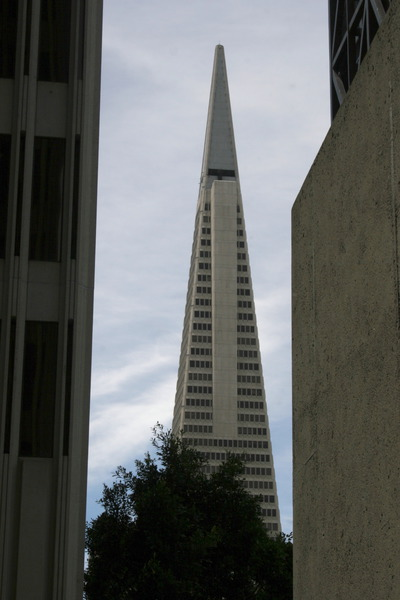ModelTop choices
Colorsyellow (score = 0.61119)
blue (score = 0.15826)
red (score = 0.14627)
Countsarray (score = 0.60478)
pair (score = 0.30132)
Face/facesface (score = 0.54169)
faces (score = 0.45831)
Geom/all angular (score = 0.63832)
triangle (score = 0.25262)
Geom/no square angular (score = 0.65672)
triangle (score = 0.26132)
Geom/no square, triangle angular (score = 0.91369)
Geom: angular, curveangular (score = 0.95763)
curve (score = 0.04237)
Geom: no angular, curvetriangle (score = 0.91157)
Graffiti/mural graffiti (score = 0.57809)
mural (score = 0.42191)
Juxtaposejuxtapose (score = 0.46356)
align (score = 0.36749)
Cases gt300 downtown (score = 0.24691)
angular (score = 0.17215)
juxtapose align (score = 0.16496)
Cases gt300 long opt downtown (score = 0.43156)
juxtapose align (score = 0.20036)
angular (score = 0.10114)
Cases gt300 longest opt downtown (score = 0.56029)
juxtapose align (score = 0.28470)
yellow (score = 0.03577)
angular (score = 0.03436)
array (score = 0.01416)ModelTop choices
Colors red (score = 0.86927)
yellow (score = 0.10955)
blue (score = 0.01950)
green (score = 0.00168)
Countsarray (score = 0.73672)
pair (score = 0.19537)
Face/facesface (score = 0.88145)
faces (score = 0.11855)
Geom/all circle (score = 0.47792)
triangle (score = 0.17521)
angular (score = 0.11576)
curve (score = 0.09584)
square (score = 0.01435)
Geom/no square circle (score = 0.39329)
triangle (score = 0.20403)
angular (score = 0.15382)
curve (score = 0.10557)
Geom/no square, triangle circle (score = 0.60697)
angular (score = 0.12103)
curve (score = 0.09558)
Geom: angular, curve curve (score = 0.56893)
angular (score = 0.43107)
Geom: no angular, curve circle (score = 0.52692)
triangle (score = 0.38199)
Graffiti/mural mural (score = 0.96716)
graffiti (score = 0.03284)
Juxtaposejuxtapose (score = 0.91897)
Cases gt300 mural (score = 0.25051)
sign (score = 0.15409)
red (score = 0.13840)
Cases gt300 long opt red (score = 0.33347)
mural (score = 0.26297)
Cases gt300 longest opt woman (score = 0.31791)
red (score = 0.19784)
face (score = 0.17757)
curve (score = 0.12499)
mural (score = 0.10240)
sign (score = 0.03846)
angular (score = 0.01052)
array (score = 0.00737)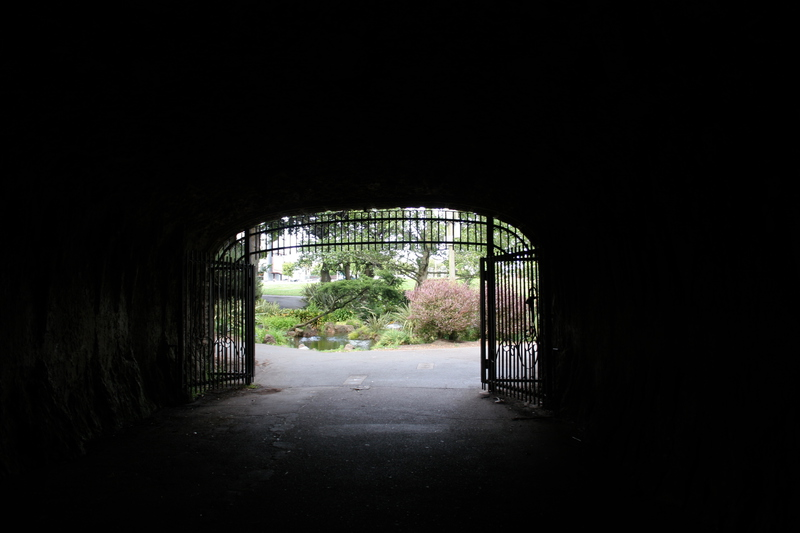ModelTop choices
Colors green (score = 0.50880)
yellow (score = 0.20146)
red (score = 0.17427)
blue (score = 0.11546)
Countsarray (score = 0.87449)
pair (score = 0.11442)
Face/facesface (score = 0.95689)
faces (score = 0.04311)
angular (score = 0.32882)
curve (score = 0.12060)
triangle (score = 0.07769)
square (score = 0.04825)
circle (score = 0.02317)
Geom/no square radiate (score = 0.41904)
angular (score = 0.36173)
curve (score = 0.12605)
triangle (score = 0.07503)
circle (score = 0.01815)
Geom/no square, triangle angular (score = 0.44917)
curve (score = 0.10503)
circle (score = 0.01625)
Geom: angular, curve angular (score = 0.85236)
curve (score = 0.14764)
Geom: no angular, curve radiate (score = 0.73884)
triangle (score = 0.14103)
Graffiti/mural mural (score = 0.72983)
graffiti (score = 0.27017)
Juxtaposeontop (score = 0.70695)
size (score = 0.11745)
Cases gt300 reflection (score = 0.12647)
angular (score = 0.09670)
array (score = 0.05481)
curve (score = 0.03991)
tree (score = 0.03799)
juxtapose align (score = 0.03726)
Cases gt300 long opt reflection (score = 0.15003)
angular (score = 0.11343)
tree (score = 0.07468)
downtown (score = 0.07069)
curve (score = 0.06577)
array (score = 0.06217)
Cases gt300 longest opt tree (score = 0.24299)
reflection (score = 0.20786)
downtown (score = 0.12085)
angular (score = 0.09329)
array (score = 0.06247)
curve (score = 0.04335)
sign (score = 0.02818)
woman (score = 0.02671)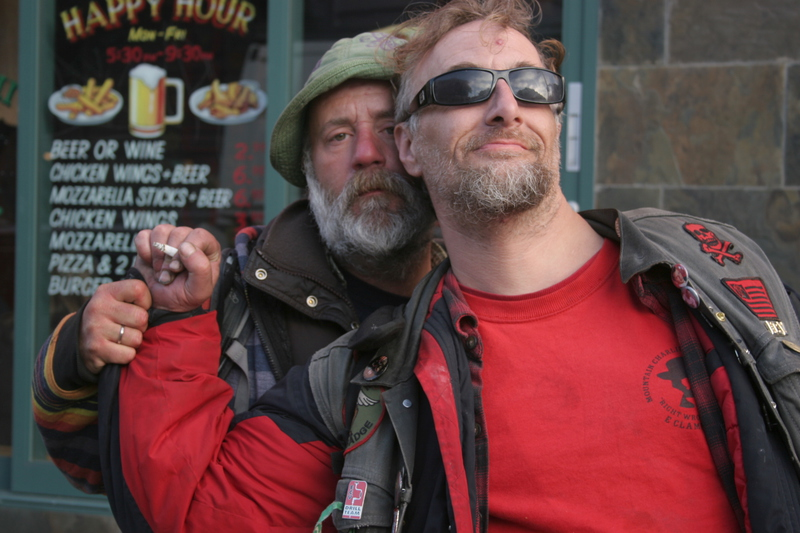ModelTop choices
Colors red (score = 0.41883)
yellow (score = 0.38110)
green (score = 0.17761)
blue (score = 0.02246)
Countspair (score = 0.95367)
Face/facesface (score = 0.88632)
faces (score = 0.11368)
Geom/all circle (score = 0.44158)
curve (score = 0.43193)
Geom/no square curve (score = 0.44450)
circle (score = 0.43765)
Geom/no square, triangle circle (score = 0.52906)
curve (score = 0.35213)
Geom: angular, curve curve (score = 0.99212)
angular (score = 0.00788)
Geom: no angular, curve circle (score = 0.91524)
Graffiti/mural graffiti (score = 0.90954)
mural (score = 0.09046)
Juxtapose juxtapose size (score = 0.39079)
juxtapose (score = 0.32190)
juxtapose align (score = 0.18765)
Cases gt300 man (score = 0.38199)
smile (score = 0.11842)
faces (score = 0.11150)
face (score = 0.11150)
pair (score = 0.09211)
woman (score = 0.06935)
Cases gt300 long opt man (score = 0.61665)
smile (score = 0.11146)
face (score = 0.08650)
faces (score = 0.04708)
sign (score = 0.04125)
pair (score = 0.03511)
woman (score = 0.02443)
Cases gt300 longest opt man (score = 0.76733)
face (score = 0.09641)
smile (score = 0.07105)
sign (score = 0.04091)
faces (score = 0.00930)ModelTop choices
Colors blue (score = 0.77404)
red (score = 0.16478)
green (score = 0.04481)
yellow (score = 0.01637)
Counts pair (score = 0.62111)
array (score = 0.28116)
trio (score = 0.09773)
Face/facesface (score = 0.73248)
faces (score = 0.26752)
Geom/all circle (score = 0.56339)
Geom/no square circle (score = 0.58437)
Geom/no square, triangle circle (score = 0.59583)
Geom: angular, curve curve (score = 0.65589)
angular (score = 0.34411)
Geom: no angular, curve circle (score = 0.63208)
Graffiti/mural mural (score = 0.98266)
graffiti (score = 0.01734)
Juxtapose juxtapose align (score = 0.14047)
juxtapose ontop (score = 0.12835)
Cases gt300 mural (score = 0.54477)
sign (score = 0.13073)
graffiti (score = 0.07619)
face (score = 0.05085)
faces (score = 0.03371)
woman (score = 0.02418)
sculpture (score = 0.01851)
man (score = 0.01586)
Cases gt300 long opt mural (score = 0.50963)
sign (score = 0.25058)
face (score = 0.08162)
faces (score = 0.04666)
woman (score = 0.02597)
man (score = 0.02009)
graffiti (score = 0.01926)
Cases gt300 longest opt sign (score = 0.83436)
mural (score = 0.06977)
face (score = 0.03206)
faces (score = 0.02475)
woman (score = 0.02273)
man (score = 0.01214)
pair (score = 0.00103)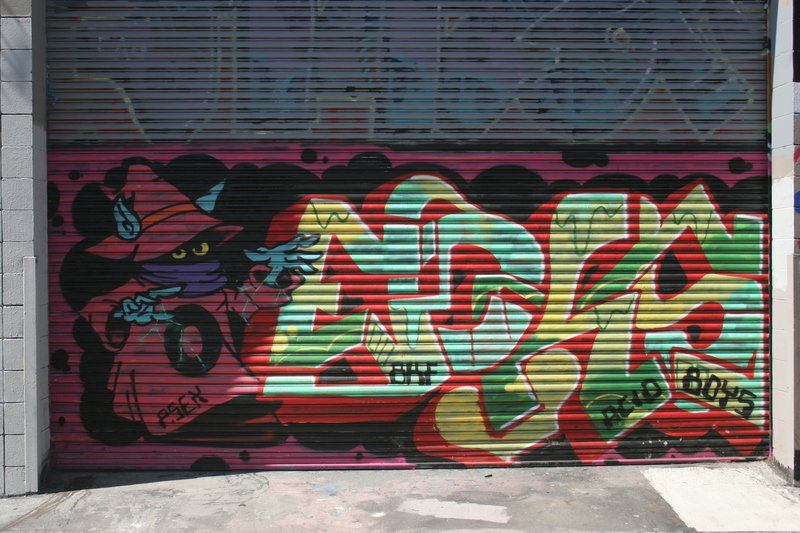ModelTop choices
Colors red (score = 0.97778)
yellow (score = 0.01651)
blue (score = 0.00371)
green (score = 0.00200)
Counts array (score = 0.82806)
pair (score = 0.17100)
trio (score = 0.00093)
Face/facesface (score = 0.89165)
faces (score = 0.10835)
circle (score = 0.26262)
square (score = 0.19681)
Geom/no square radiate (score = 0.59929)
circle (score = 0.26423)
Geom/no square, triangle radiate (score = 0.56058)
circle (score = 0.36628)
Geom: angular, curve curve (score = 0.69182)
angular (score = 0.30818)
Geom: no angular, curve radiate (score = 0.45189)
circle (score = 0.34247)
square (score = 0.17949)
Graffiti/mural graffiti (score = 0.62841)
mural (score = 0.37159)
Juxtapose juxtapose (score = 0.39611)
juxtapose ontop (score = 0.33690)
juxtapose texture (score = 0.23557)
Cases gt300 graffiti (score = 0.50994)
mural (score = 0.29014)
red (score = 0.08554)
sign (score = 0.03895)
orange (score = 0.00918)
pink (score = 0.00712)
yellow (score = 0.00673)
woman (score = 0.00644)
face (score = 0.00550)
Cases gt300 long opt graffiti (score = 0.57802)
mural (score = 0.18495)
red (score = 0.14777)
flowers (score = 0.01842)
sign (score = 0.01335)
face (score = 0.01082)
orange (score = 0.01042)
woman (score = 0.00768)
pink (score = 0.00591)
Cases gt300 longest opt red (score = 0.68922)
graffiti (score = 0.25977)
mural (score = 0.04059)
flowers (score = 0.00389)
face (score = 0.00303)
sign (score = 0.00101)
orange (score = 0.00093)
woman (score = 0.00041)
man (score = 0.00023)
pink (score = 0.00019)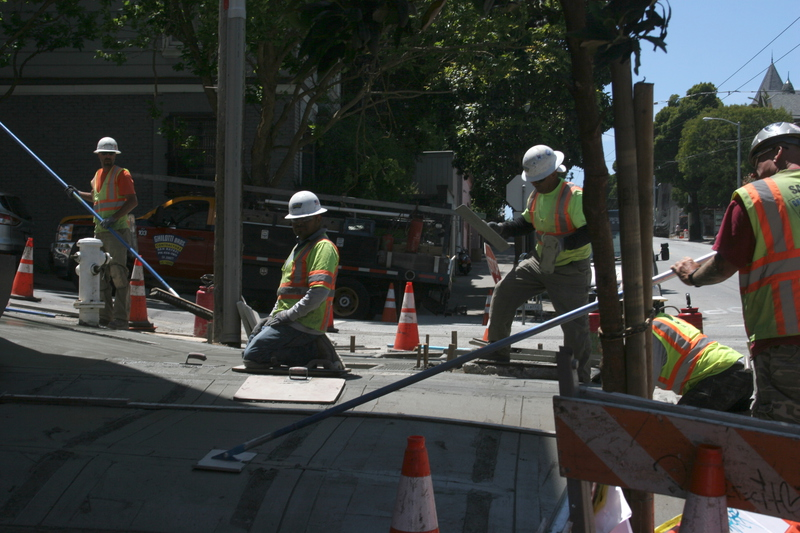ModelTop choices
Colors red (score = 0.57240)
yellow (score = 0.22224)
green (score = 0.15980)
blue (score = 0.04556)
Counts pair (score = 0.90147)
trio (score = 0.06785)
array (score = 0.03068)
Face/facesface (score = 0.79100)
faces (score = 0.20900)
Geom/all angular (score = 0.90688)
Geom/no square angular (score = 0.93529)
Geom/no square, triangle angular (score = 0.89503)
Geom: angular, curve angular (score = 0.98396)
curve (score = 0.01604)
Geom: no angular, curve circle (score = 0.77155)
Graffiti/mural graffiti (score = 0.84843)
mural (score = 0.15157)
Juxtapose juxtapose (score = 0.55615)
juxtapose opposite (score = 0.20296)
juxtapose size (score = 0.11106)
juxtapose align (score = 0.10795)
Cases gt300 man (score = 0.27430)
pair (score = 0.20783)
orange (score = 0.15351)
pink (score = 0.04409)
angular (score = 0.03886)
red (score = 0.03880)
yellow (score = 0.03633)
costume (score = 0.02792)
face (score = 0.02341)
hang (score = 0.02126)
woman (score = 0.01767)
Cases gt300 long opt man (score = 0.48577)
pair (score = 0.19973)
orange (score = 0.09606)
costume (score = 0.04682)
red (score = 0.04046)
hang (score = 0.03596)
face (score = 0.02329)
woman (score = 0.02002)
array (score = 0.01077)
angular (score = 0.00696)
faces (score = 0.00580)
Cases gt300 longest opt pair (score = 0.57577)
man (score = 0.33279)
orange (score = 0.01921)
hang (score = 0.01767)
costume (score = 0.01674)
woman (score = 0.01397)
face (score = 0.00997)
red (score = 0.00456)
array (score = 0.00446)
pink (score = 0.00162)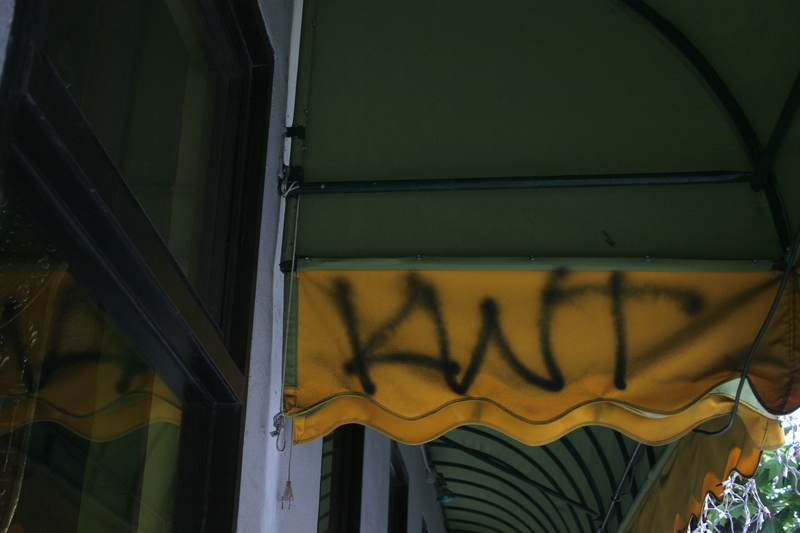ModelTop choices
Colors
yellow (score = 0.93183)
green (score = 0.04671)
red (score = 0.01968)
blue (score = 0.00178)
Counts array (score = 0.87711)
pair (score = 0.11583)
trio (score = 0.00706)
Face/facesface (score = 0.93992)
faces (score = 0.06008)
Geom/all curve (score = 0.54647)
triangle (score = 0.12134)
circle (score = 0.10313)
Geom/no square curve (score = 0.58894)
triangle (score = 0.10396)
Geom/no square, triangle curve (score = 0.67266)
Geom: angular, curve curve (score = 0.87566)
angular (score = 0.12434)
Geom: no angular, curve triangle (score = 0.39984)
circle (score = 0.29224)
Graffiti/mural mural (score = 0.51593)
graffiti (score = 0.48407)
Juxtapose juxtapose ontop (score = 0.27392)
juxtapose opposite (score = 0.22061)
juxtapose (score = 0.21500)
juxtapose texture (score = 0.15487)
Cases gt300 yellow (score = 0.19636)
reflection (score = 0.11763)
hang (score = 0.11245)
mural (score = 0.10110)
red (score = 0.05969)
graffiti (score = 0.04734)
Cases gt300 long opt yellow (score = 0.59058)
curve (score = 0.07078)
graffiti (score = 0.05900)
reflection (score = 0.04290)
orange (score = 0.02897)
mural (score = 0.02599)
Cases gt300 longest opt yellow (score = 0.82725)
curve (score = 0.10109)
man (score = 0.01327)
graffiti (score = 0.01245)
face (score = 0.01015)
reflection (score = 0.00836)
array (score = 0.00759)
mural (score = 0.00394)ModelTop choices
Colors
red (score = 0.41308)
blue (score = 0.24517)
yellow (score = 0.19776)
green (score = 0.14399)
Counts array (score = 0.98655)
Face/facesface (score = 0.98149)
faces (score = 0.01851)
Geom/all circle (score = 0.54992)
curve (score = 0.12666)
Geom/no square circle (score = 0.52875)
curve (score = 0.15973)
Geom/no square, triangle circle (score = 0.55429)
curve (score = 0.17587)
Geom: angular, curve curve (score = 0.82266)
angular (score = 0.17734)
Geom: no angular, curve circle (score = 0.70552)
Graffiti/mural graffiti (score = 0.54836)
mural (score = 0.45164)
Juxtapose juxtapose opposite (score = 0.69507)
juxtapose align (score = 0.10592)
Cases gt300 array (score = 0.14068)
orange (score = 0.09043)
pink (score = 0.06697)
sculpture (score = 0.05755)
red (score = 0.04813)
face (score = 0.04804)
Cases gt300 long opt array (score = 0.48226)
pink (score = 0.13873)
sculpture (score = 0.05713)
man (score = 0.03878)
orange (score = 0.03651)
face (score = 0.03533)
Cases gt300 longest opt pink (score = 0.41445)
array (score = 0.35484)
curve (score = 0.09533)
sculpture (score = 0.04074)
face (score = 0.02953)
man (score = 0.01747)
sign (score = 0.01112)
tree (score = 0.00858)

Phobrain pair matches (click on a photo to see another pair). Could a neural net be trained to spot what is in common?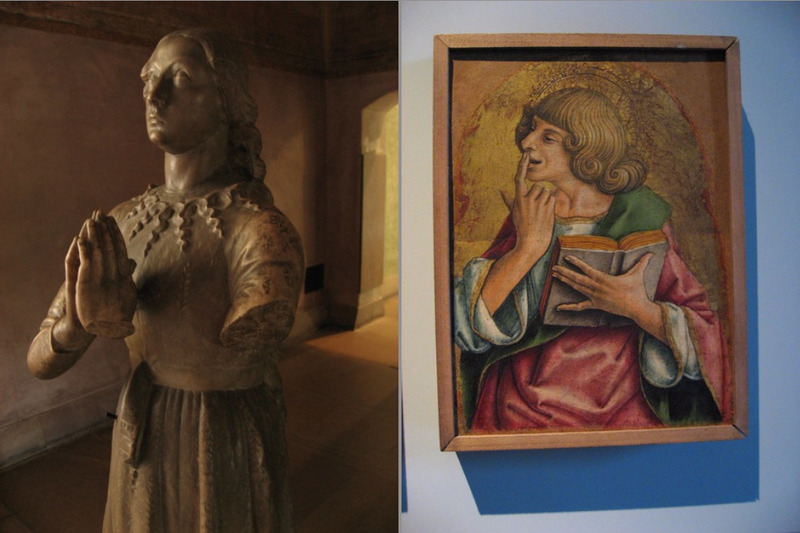Photos: Raf & Skot